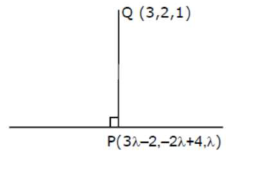# Let L be a line obtained from the intersection

Question:

Let $\mathrm{L}$ be a line obtained from the intersection of two planes $x+2 y+z=6$ and $y+2 z=4$. If point $P(\alpha, \beta, \gamma)$ is the foot of perpendicular from $(3,2,1)$ on $L$, then the value of $21(\alpha+\beta+\gamma)$ equals :

1. (1) 142

2. (2) 68

3. (3) 136

4. (4) 102

Correct Option: , 4

Solution:

Dr's of line $\left|\begin{array}{lll}\hat{i} & \hat{j} & \hat{k} \\ 1 & 2 & 1 \\ 0 & 1 & 2\end{array}\right|=3 \hat{i}-2 \hat{j}+\hat{k}$

Dr/s: $-(3,-2,1)$

Points on the line $(-2,4,0)$ Equation of the line $\frac{x+2}{3}=\frac{y-4}{-2}=\frac{z}{1}=\lambda$Dr's of $\mathrm{PQ} ; 3 \lambda-5,-2 \lambda+2 . \lambda-1$

Dr's of y lines are $(3,-2,1)$ Since $\mathrm{PQ} \perp$ line $3(3 \lambda-5)-2(-2 \lambda+2)+1(\lambda-1)=0$

$\lambda=\frac{10}{7}$

$P\left(\frac{16}{7}, \frac{8}{7}, \frac{10}{7}\right)$

$21(\alpha+\beta+\gamma)=21\left(\frac{34}{7}\right)=102$